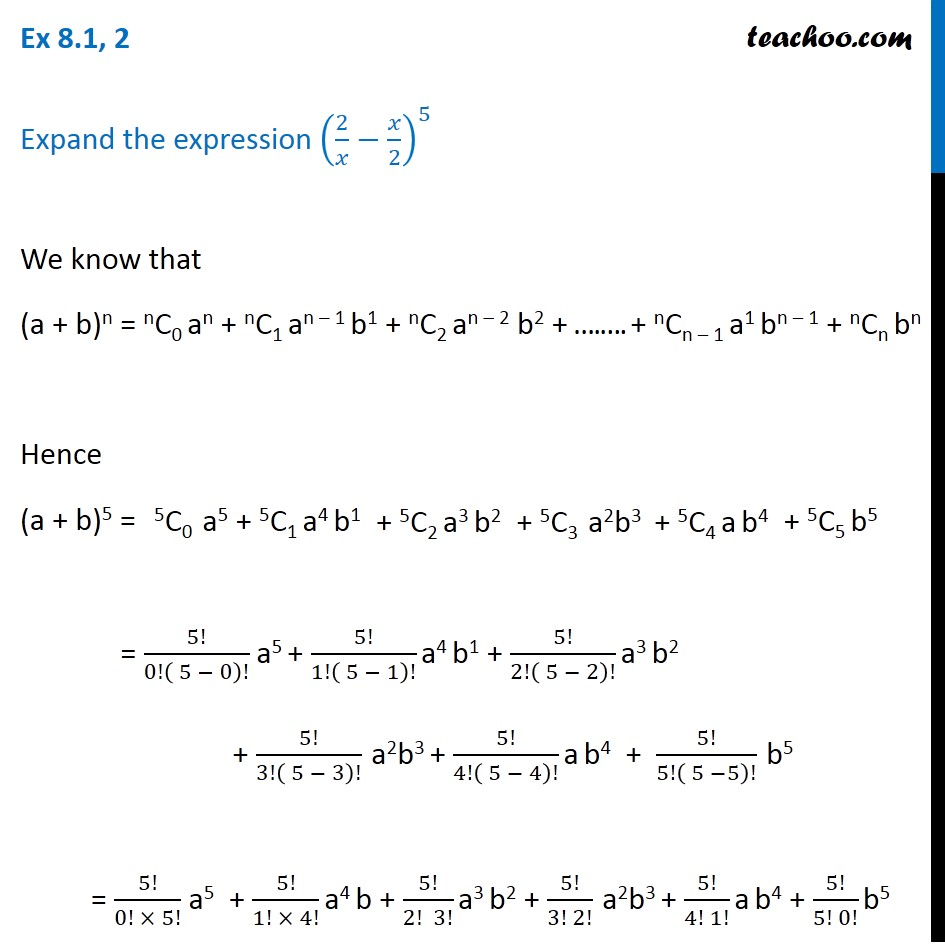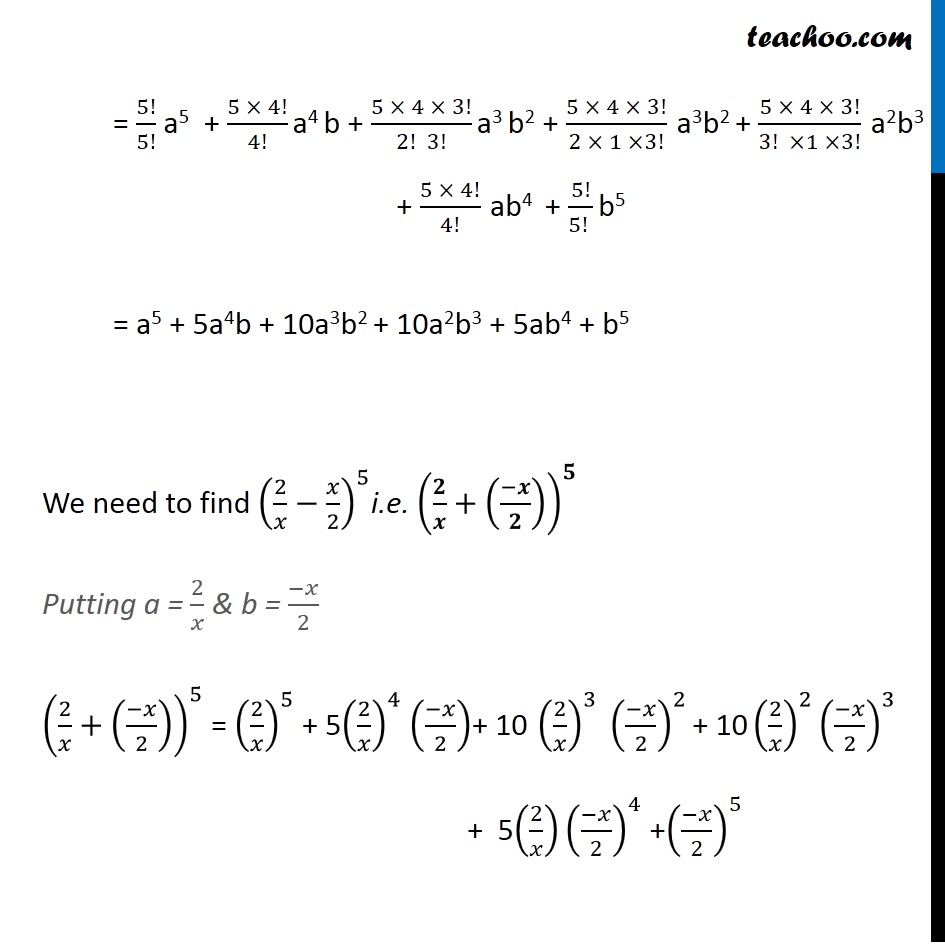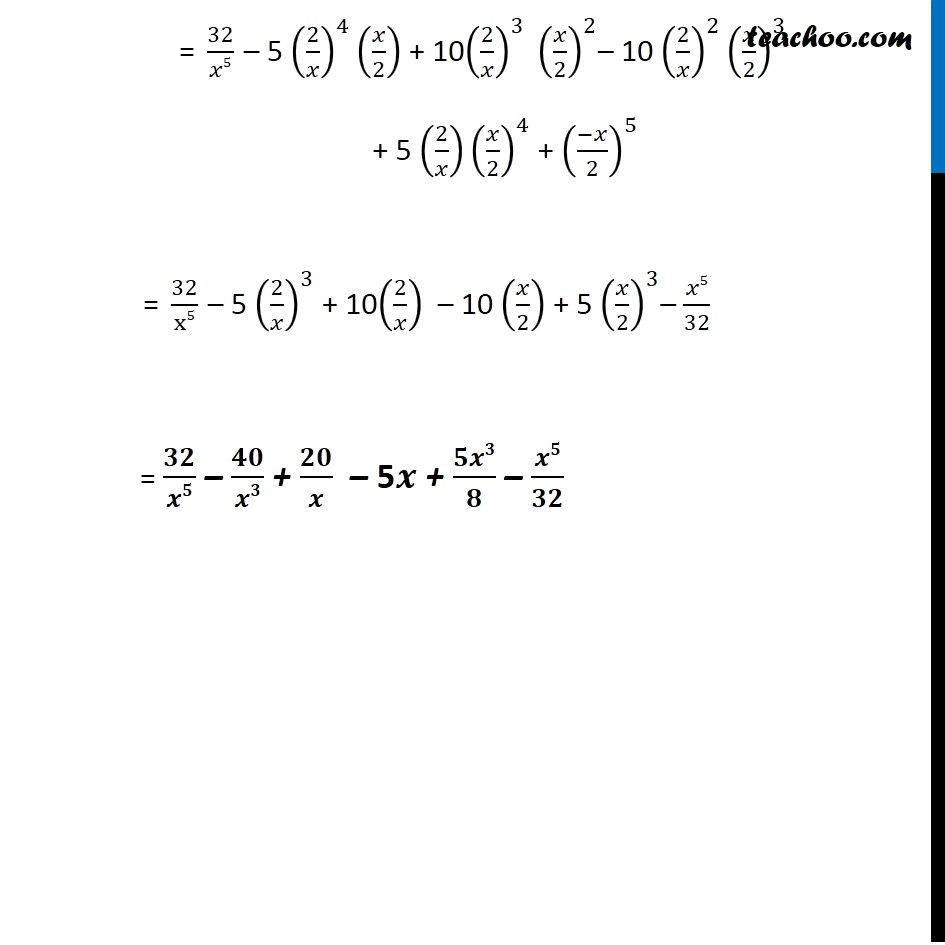Expansion

Chapter 8 Class 11 Binomial Theorem (Deleted)
Concept wise### Transcript

Ex 8.1, 2 Expand the expression (2/𝑥−𝑥/2)^5 We know that (a + b)n = nC0 an + nC1 an – 1 b1 + nC2 an – 2 b2 + ….…. + nCn – 1 a1 bn – 1 + nCn bn Hence (a + b)5 = = 5!/0!( 5 − 0)! a5 + 5!/1!( 5 − 1)! a4 b1 + 5!/2!( 5 − 2)! a3 b2 + 5!/3!( 5 − 3)! a2b3 + 5!/4!( 5 − 4)! a b4 + 5!/5!( 5 −5)! b5 = 5!/(0! × 5!) a5 + 5!/(1! × 4!) a4 b + 5!/(2! 3!) a3 b2 + 5!/(3! 2!) a2b3 + 5!/(4! 1!) a b4 + 5!/(5! 0!) b5 = 5!/5! a5 + (5 × 4!)/4! a4 b + (5 × 4 × 3!)/(2! 3!) a3 b2 + (5 × 4 × 3!)/(2 × 1 ×3!) a3b2 + (5 × 4 × 3!)/(3! ×1 ×3!) a2b3 + (5 × 4!)/4! ab4 + 5!/(5! ) b5 = a5 + 5a4b + 10a3b2 + 10a2b3 + 5ab4 + b5 We need to find (2/𝑥−𝑥/2)^5i.e. (𝟐/𝒙+((−𝒙)/𝟐))^𝟓 Putting a = 2/𝑥 & b = (−𝑥)/2 (2/𝑥+((−𝑥)/2))^5 = (2/𝑥)^5 + 5(2/𝑥)^4 ((−𝑥)/2)+ 10 (2/𝑥)^3 ((−𝑥)/2)^2 + 10 (2/𝑥)^2 ((−𝑥)/2)^3 + 5(2/𝑥) ((−𝑥)/2)^4 +((−𝑥)/2)^5 = 32/𝑥5 – 5 (2/𝑥)^4 (𝑥/2) + 10(2/𝑥)^3 (𝑥/2)^2– 10 (2/𝑥)^2 (𝑥/2)^3 + 5 (2/𝑥) (𝑥/2)^4 + ((−𝑥)/2)^5 = 32/x5 – 5 (2/𝑥)^3 + 10(2/𝑥) – 10 (𝑥/2) + 5 (𝑥/2)^3– 𝑥5/32 = 𝟑𝟐/𝒙𝟓 – 𝟒𝟎/𝒙𝟑 + 𝟐𝟎/𝒙 – 5𝒙 + 𝟓𝒙𝟑/𝟖 – 𝒙𝟓/𝟑𝟐